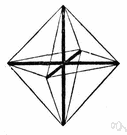# affine transformation

(redirected from Affinely independent)
Also found in: Thesaurus, Encyclopedia.
ThesaurusAntonymsRelated WordsSynonymsLegend:
 Noun 1affine transformation - (mathematics) a transformation that is a combination of single transformations such as translation or rotation or reflection on an axismath, mathematics, maths - a science (or group of related sciences) dealing with the logic of quantity and shape and arrangementtransformation - (mathematics) a function that changes the position or direction of the axes of a coordinate system
References in periodicals archive ?
A set {[v.sub.0], ..., [v.sub.n]} of vertexes is affinely independent if and only if the vectors [v.sub.1] - [v.sub.0], ..., [v.sub.n] - [v.sub.0] are linearly independent.
For simplicity, we often denote the simplex spanned by a set {[v.sub.0], ..., [v.sub.n]} of affinely independent vertexes as ([v.sub.0], ..., [v.sub.n]).
Let S = ([s.sub.0], ..., [s.sub.p]) and T = ([t.sub.0], ..., [t.sub.q]) be simplexes whose combined sets of vertexes are affinely independent. Then the join of S and T, denoted S [multiplied by] T is the simplex ([s.sub.0], ..., [s.sub.p], [t.sub.0], ..., [t.sub.q]).

Site: Follow: Share:
Open / Close